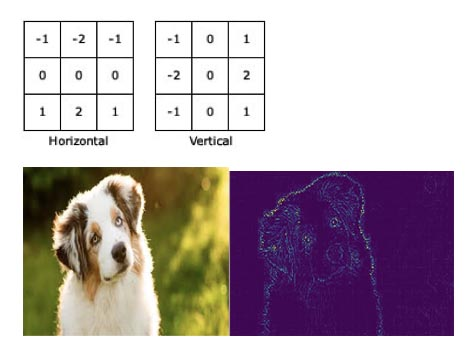# Understanding how filters are created in torch.nn.Conv2d

Hey everyone, I have a question about connecting the convolution in this picture to the `torch.nn.Conv2d` function. So to recapitulate the convolution step, I’d need to set the `out_channels = 2` and `kernel_sizes = [2, 3, 4]`. My question is how are the values in the filter matrices calculated? I assume the two filters with size 2 aren’t the same. Any help is appreciated!

Just to add another point to this to make it more clear, when reading about CNN for images, I come across these pre-defined filters (see below). How come there is no option for that in `torch.nn.Conv2d`?You can manually set the parameters in a layer (e.g.,

``````mylayer = torch.nn.Conv2d(1,1,1,1)
mylayer.weight = ...
``````

However, this is typically not done because the layers are trained with gradient descent. Predefined filters are more of a hallmark of classical computer vision for tasks like line and corner detection but typically convolutional layers in neural networks are trained from randomly initialized parameters.

1 Like

I gotcha, that totally makes sense that the values in the filter matrices are the weights that are updated through backprop.

If it helps, I’ve implemented the model. Let me know if anything’s unclear…or you spot any errors :).

2 Likes